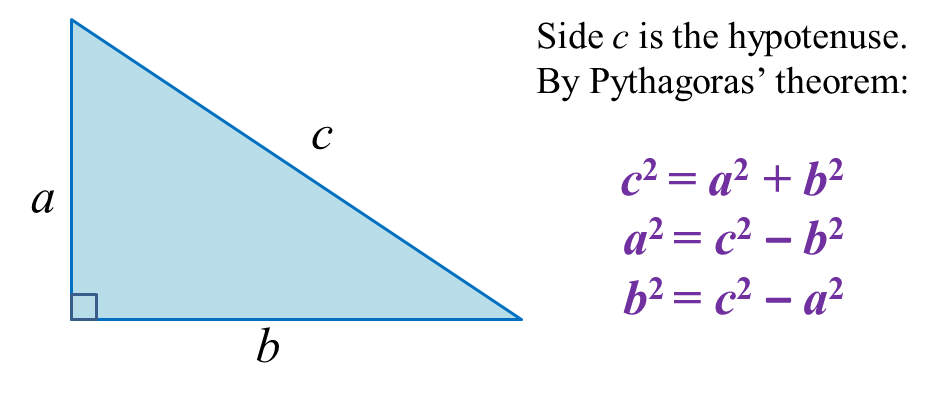# 13.1 The Pythagoras’ Theorem

13.1 The Pythagoras’ Theorem

13.1.1 The Pythagoras’ Theorem
1. In a right-angled triangle, the hypotenuse is the longest side of the triangle.
2. Pythagoras’ Theorem:

 In a right-angled triangle, the square   of the hypotenuse is equal to the sum   of the squares of the other two sides.Example 1:Solution:
$\begin{array}{l}{x}^{2}={5}^{2}+{12}^{2}\\ \text{}=25+144\\ \text{}x=\sqrt{169}\\ \text{}=13\end{array}$

Example 2:Solution:
$\begin{array}{l}{x}^{2}={15}^{2}-{9}^{2}\\ \text{}=225-81\\ \text{}x=\sqrt{144}\\ \text{}=12\end{array}$

3. Pythagorean triples
are three whole numbers that form the sides of a right-angled triangle.

Example:
(a) 3, 4, 5
(b) 6, 8, 10
(c) 5, 12, 13
(d) 8, 15, 17
(e) 9, 12, 15

13.1.2 The Converse of the Pythagoras’ Theorem

 In a triangle, if the sum of the squares of the two sides   is equal to the square of the longest side, then the angle   opposite the longest side is a right angle.